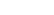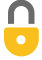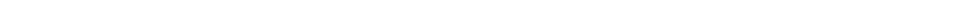Problem 5
Q

# A British thermal unit (Btu) is a unit of heat in the British system of units. One Btu is defined as the heat needed to raise 1 lb of water by $1 \textrm{ F}^\circ$. Show that 1 Btu = 0.252 kcal = 1056 J.A
$1055 \textrm{ J, } 0.252 \textrm{ kcal}$In order to watch this solution you need to have a subscription.VIDEO TRANSCRIPT

This is Giancoli Answers with Mr. Dychko. 1 BTU is defined as the heat needed to raise 1 pound of water by 1 Fahrenheit degree. So, we can take a pound of water and multiply it by 1 kilogram for every 2.2046 pounds and this makes the pounds cancel. And we're using this formula here because, you know, doesn't matter what your units are, heat transfer, Q, can be in BTU or joules or whatever you like, calories and so on. It's always going to equal mass times specific heat capacity times a change a temperature. So, we're told the mass here is 1 pound and the substance is water. And so it's specific heat capacity, we have to multiply by 4,186 joules per kilogram Celsius degree. But since we have joules on the top here and kilogram Celsius degree in the bottom, we need to convert these pounds into kilograms. And then we also need to convert this Fahrenheit degree into Celsius degrees. So, there's... For every 5/9 of a Celsius degree there's 1 Fahrenheit degree and we know that based on this conversion formula from Fahrenheit into Celsius, that, you know, that's the slope of this graph here. We saw this formula in the last chapter. And you can expand or, you know, distribute this 5/9 into the bracket there and you have 5/9 times Fahrenheit minus 32 times 5/9. But it's the slope that's important here, and this means in that for every 1 degree rise in Fahrenheit, you get 5/9 of a degree rise in Celsius. And so that's a conversion factor between Celsius degrees and Fahrenheit degrees and the Fahrenheits cancel, and sort of the Celsius, and then the kilograms are cancelling. And now we've ended up converting BTU into joules. And so 1 BTU is 1,055 joules. And then multiplying by 1 kilocalorie for every 4.186 times 10 to the 3 joules, gives us 0.252 kilocalories.

Giancoli Answers, including solutions and videos, is copyright © 2009-2023 Shaun Dychko, Vancouver, BC, Canada. Giancoli Answers is not affiliated with the textbook publisher. Book covers, titles, and author names appear for reference purposes only and are the property of their respective owners. Giancoli Answers is your best source for the 7th and 6th edition Giancoli physics solutions.